# Quantitative Aptitude Quiz for Prelims Exams- SBI & IBPS 2020- 29th November

Q1. The price of petrol is increased by 25% by what percent should a car owner reduce the consumption of petrol so that his expenditure on petrol remains constant?
(a) 18%
(b) 16%
(c) 15%
(d) 20%
(e) 14%

Q2. Neha scored 37% marks and failed by 78 marks. If she scored 42% marks, she fails by 48 marks. Find by how much marks would she fail or pass if she scored 48% marks?
(a) 16 marks,fail
(b) 12 marks,fail
(c) 10 marks,pass
(d) 18 marks,pass
(e) 25 marks, fail

Q3. Rohit scores 84 in Maths while 79 in Science. Karan scores 85 in Maths. If average score of Karan is 6 more than average score of Rohit in both the subjects. Find the marks scored by Karan in Science.
(a) 90
(b) 85
(c) 87.5
(d) 81.5
(e) 85.5

Q4. Average of sum of four consecutive even numbers is 10 more than the average of sum of three consecutive odd numbers. If largest even number is twice the smallest odd number. Find average of all seven numbers.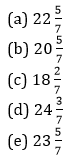Q5. Ratio of age of Ravi to Vicky, 4 years ago was 5: 6, while ratio of present age of Rocky to that of Vicky is 5: 4. If 2 years later sum of age of Ravi and Rocky will be 63 years, then find the difference between present age of Ravi and Vicky?
(a) 4 years
(b) 2 years
(c) 8 years
(d) 6 years
(e) 5 years

Q6. Three years ago, average age of ‘Amit’, ‘Bittu’ and ‘Chitu’  is 27 years. Four years hence, ratio of Amit and Chitu’s age is 7 : 10. If Bittu is 6 years younger than Chitu, then find present age of ‘Amit’?
(a) 24 years
(b) 27 years
(c) 30 years
(d) 28 years
(e) 36 years

Q7. In first mixture, ratio of milk to water is 7: 9. After adding 24 ltr of second mixture (having ratio of water to milk 3: 5) in the first mixture, the quantity of milk and water becomes equal in the final mixture. Find the total quantity of milk in the final mixture.
(a) 28 ltr
(b) 30 ltr
(c) 36 ltr
(d) 32 ltr
(e) 24 ltr

Q8.  8 kg of type A wheat, at the rate of Rs. 60 per kg mixed with 12 kg of type B wheat, at the rate of Rs. X per kg. If the price of resulting mixture is Rs. 67.2 per kg, then find at what price should per kg type B wheat sold to make a profit of 25%?
(a) 84 Rs.
(b) 96 Rs.
(c) 104 Rs.
(d) 90 Rs.
(e) 78 Rs.

Q9. Amount of Rs. 8000 is lent at simple interest in two parts at 20% and 10% respectively. If after one year he will get Rs. 1150 as interest then find amount which was lent at 20% per annum.
(a) Rs.3000
(b) Rs.5000
(c) Rs.3500
(d) Rs.4500
(e) Rs. 4200

Q10.  A train ‘P’ crosses a pole in 6.75 sec and a 240-meter-long platform in 15.75 sec. If train ‘Q’ which is 120-meter-long running in same direction crosses train ‘P’ in 45 sec, then find time required by train ‘Q’ to cross train ‘P’ running in opposite direction?
(a)  6sec
(b) 7sec
(c) 5 sec
(d) 9 sec
(e) 10 sec

Q11. A & B working together can complete a piece of work in 72 days, B & C working together can complete the same work in 90 days and A & C working together can complete the same work in 75 days. If A, B & C starts working together, then find in how many days will the same work be completed?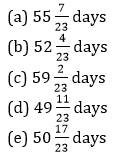Q12. Shubham work for 5 days and remaining work was completed by Harvinder in 9 days. If Harvinder work for 12 days then remaining work was completed by Shubham in 3 days, then find how much time Harvinder will take to complete the work alone.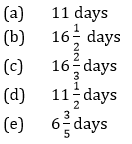Q13. A man sees a train passing over a bridge of length 1 km. The length of the train is half of the length of bridge. If the train passes the bridge in 2 minutes then find the speed of the train?
(a) 30 kmph
(b) 45 kmph
(c) 50 kmph
(d) 60 kmph
(e) 54 kmph

Q14.    The ratio of length of two train A and B are 7 : 11. If these two train crosses each other while travelling in same direction in 144 sec given that speed of train A and B is 99 kmph and 63 kmph respectively. Then find the difference between length of both train.
(a) 320 m
(b) 200 m
(c) 180 m
(d) 360 m
(e) 400 m

Q15. Ratio of still water speed of a boat to speed of current is 3 : 1. It covers 45 km in downstream in X hours and 27 km in upstream in (X+ ½)  hours. Find speed of boat in upstream.
(a) 6 km/hr
(b) 4 km/hr
(c) 5 km/hr
(d) 8 km/hr
(e) None of these

Practice More Questions of Quantitative Aptitude for Competitive Exams:

Quantitative Aptitude for Competitive Exams
###### Prelims Quiz Study Plan for SBI & IBPS Exams 2020

Solutions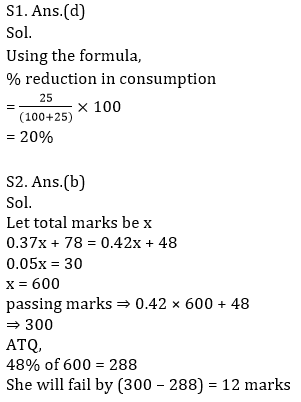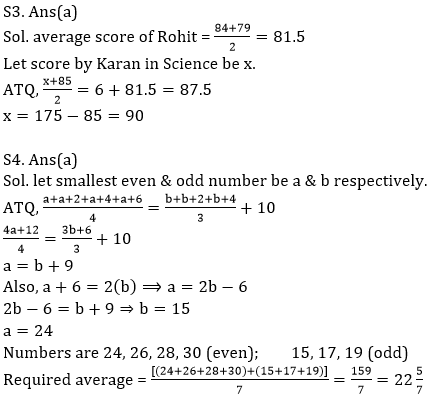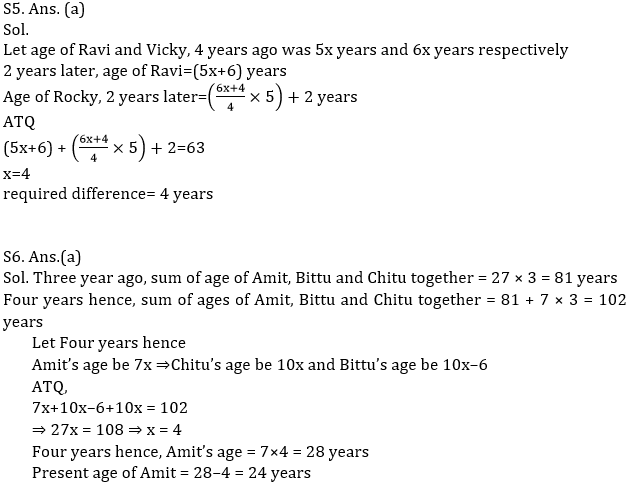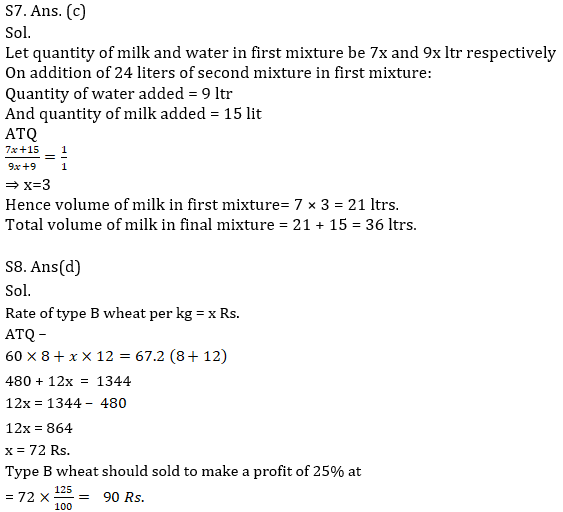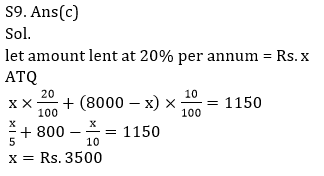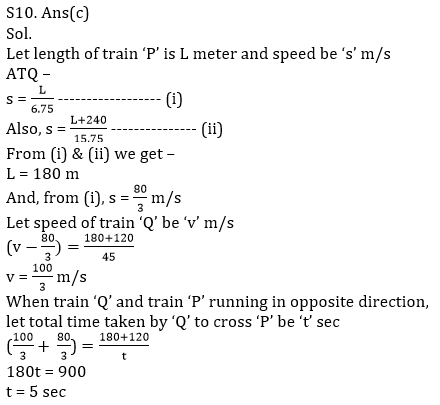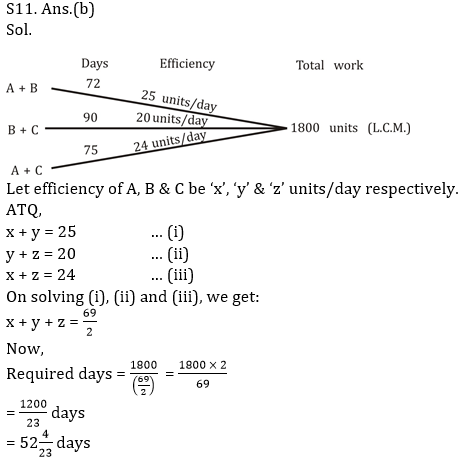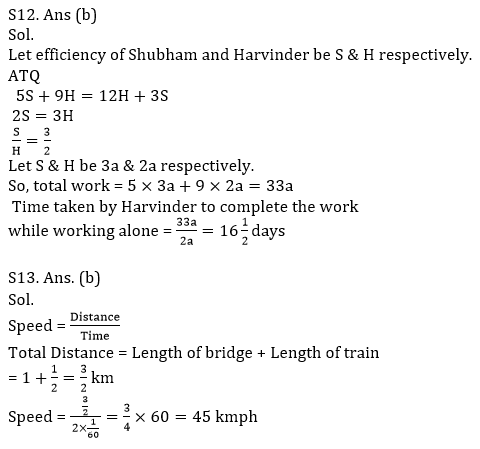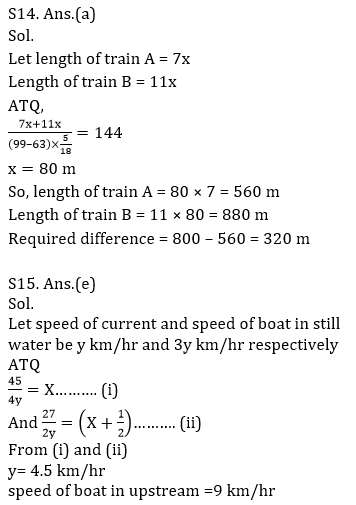Practice with Crash Course and Online Test Series for IBPS Clerk Prelims: